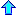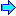# Research Interests

Numerical Analysis, Partial Differential Equations, Scientific Computing, Applied and Industrial Mathematics, Inter-Disciplinary Research.

In particular I am interested in numerical approximation of solutions of partial differential equations (using the finite element, boundary element, finite volume, and multigrid methods). I have experience analyzing these methods and developing algorithms based on these methods for approximating solutions of partial differential equations arising in fluid dynamics. I have developed and studied algorithms for approximating solutions of the Navier Stokes equations and the equations of Magnetohydrodynamics.

Recently I have been involved in several inter-disciplinary research projects. I have collaborated with chemists, physicists, and engineers.HomeBackgroundajm@math.auburn.edu